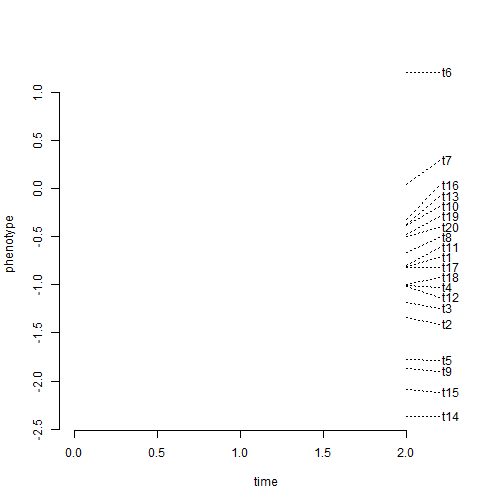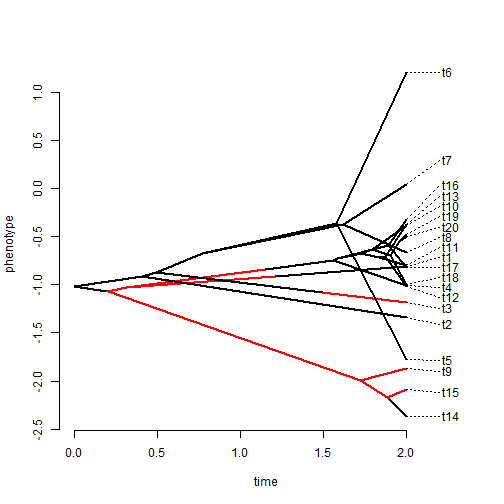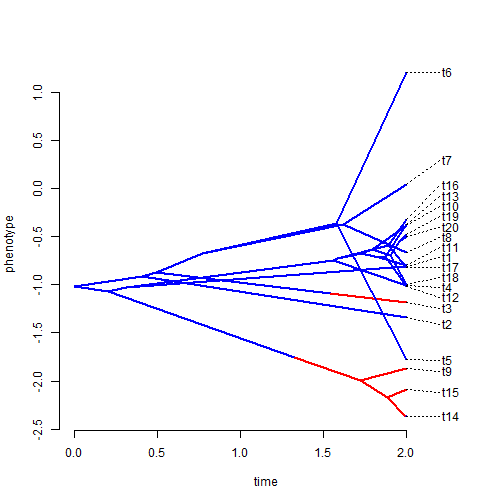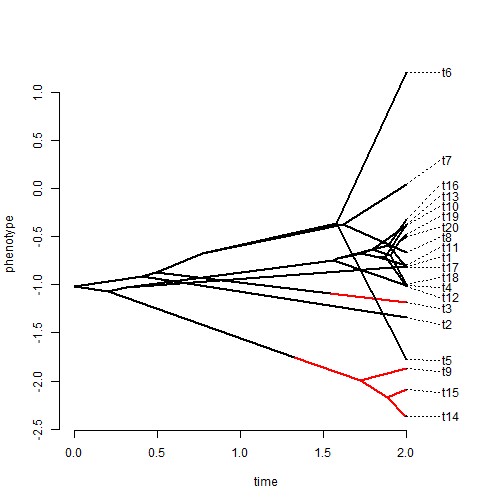## Thursday, August 20, 2015

### Fix to default colors in phenogram

https://raw.githubusercontent.com/liamrevell/phytools/master/R/phenogram.R

A phytools reader reported the following issue in using the phytools traitgram function, `phenogram`, to plot a tree with a mapped discrete character:

``````library(phytools)
``````
``````## Loading required package: ape
``````
``````tree<-pbtree(n=20,scale=2)
Q=matrix(c(-1,1,1,-1),2,2)
rownames(Q)<-colnames(Q)<-LETTERS[1:2]
x1<-sim.history(tree,Q,anc="A")\$states
``````
``````## Done simulation(s).
``````
``````x1
``````
``````## t14 t15  t9  t1 t19 t20 t18 t10 t12 t13 t11 t16 t17  t4  t2  t3  t5  t6
## "A" "B" "B" "A" "A" "A" "A" "A" "A" "A" "A" "A" "A" "A" "A" "B" "A" "A"
##  t7  t8
## "A" "A"
``````
``````y<-fastBM(tree)
t1<-make.simmap(tree,x1)
``````
``````## make.simmap is sampling character histories conditioned on the transition matrix
## Q =
``````
``````##            A          B
## A -0.2959494  0.2959494
## B  0.2959494 -0.2959494
``````
``````## (estimated using likelihood);
## and (mean) root node prior probabilities
## pi =
``````
``````##   A   B
## 0.5 0.5
``````
``````## Done.
``````
``````phenogram(t1,y)
``````By contrast, if we use a numerically indexed discrete character, we don't face the same issue:

``````x2<-sapply(x1,function(x) if(x=="A") "1" else "2")
t2<-make.simmap(tree,x2)
``````
``````## make.simmap is sampling character histories conditioned on the transition matrix
## Q =
``````
``````##            1          2
## 1 -0.2959494  0.2959494
## 2  0.2959494 -0.2959494
``````
``````## (estimated using likelihood);
## and (mean) root node prior probabilities
## pi =
``````
``````##   1   2
## 0.5 0.5
``````
``````## Done.
``````
``````phenogram(t2,y)
``````The issue is not that deep. In fact, it can be circumvented if we are willing to just use the argument `colors` (as we would in `plotSimmap`) to set the colors of our mapped discrete trait. E.g.,

``````colors<-setNames(c("blue","red"),LETTERS[1:2])
colors
``````
``````##      A      B
## "blue"  "red"
``````
``````phenogram(t1,y,colors=colors)
``````I have nonetheless fixed this issue so that we will get the mapped discrete state colored by default if no colors are specified.

``````source("https://raw.githubusercontent.com/liamrevell/phytools/master/R/phenogram.R")
phenogram(t1,y)
``````phytools is now on GitHub, so in fact this update can be installed automatically in a fresh R session using the devtools package as follows:

``````## don't run
library(devtools)
install_github("liamrevell/phytools")
``````

That's all folks.